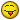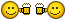# LL - Label Line changing code to Label point with its own layer name## Recommended PostsHi,

I am trying to find a way to label points with its own layer name like the attached lisp commando. It does it with line/polylines.

Also I am trying to find a way to do this with multiple points, therefore the rotation of the imported mtext does not need to be aligned.

Could anyone help me find a way to edit the code downstairs or help me with a new code?

(defun alg-ang    (obj pnt)
(angle '(0. 0. 0.)
(vlax-curve-getfirstderiv
obj
(vlax-curve-getparamatpoint
obj
pnt
)
)
)
)

(defun C:LL (/ *error* acsp adoc ang fld midp mtx rot sset txtpt)
(defun *error*  (msg)
(if
(vl-position
msg
'("console break"
"Function cancelled"
"quit / exit abort"
)
)
(princ "Error!")
(princ msg)
)
(vla-endundomark
(vla-get-activedocument
)
)
(princ)
)

(vla-get-activedocument
)
)
)
(if (and
(= (getvar "tilemode") 0)
(= (getvar "cvport") 1)
)
(setq acsp (vla-get-paperspace adoc))
(setq acsp (vla-get-modelspace adoc))
)
(vla-startundomark
)
(if (setq sset (ssget "_:L" (list (cons 0 "*LINE,ARC,CIRCLE,ELLIPSE"))))
(foreach obj
(mapcar 'vlax-ename->vla-object
(vl-remove-if 'listp
(mapcar 'cadr (ssnamex sset))))
(if (not (eq "AcDbArc"  (vla-get-objectname obj)))
(setq midp (vlax-curve-getclosestpointto obj
(vlax-curve-getpointatparam obj
( / (- (vlax-curve-getEndParam obj)
(vlax-curve-getStartParam obj)) 2))
)
)
(setq midp (vlax-curve-getclosestpointto obj
(vlax-curve-getpointatdist obj
( / (vla-get-arclength obj) 2)))
)
)

(setq ang (alg-ang obj midp))

(if (> pi ang (/ pi 2))
(setq ang (+ ang pi))
)
(if (> (* pi 1.5) ang pi)
(setq ang (+ ang pi))
)
(setq rot (+ ang (/ pi 2)))

(setq txtpt (polar midp rot
(if (zerop (getvar "dimtxt"))
0.1
(/ (getvar "dimtxt") 2)))
)

(setq fld (strcat "%<\\AcObjProp Object(%<\\_ObjId "
(itoa (vla-get-objectid obj))
">%).Layer>%")

)

(setq mtx (vlax-invoke
acsp 'AddMText midp 0.0 fld)
)
(vlax-put mtx 'AttachmentPoint
8

)
(vlax-put mtx 'InsertionPoint
txtpt
)
(vlax-put mtx 'Rotation
ang
)
)
)
(princ)
)
(princ "\n\t\t\tType LL to label curves with layer name\t")
(prin1)

##### Share on other sitesLike this?

Command LL

```

(defun M-Text (pt str ht)
(entmakex (list (cons 0 "MTEXT")
(cons 100 "AcDbEntity")
(cons 100 "AcDbMText")
(cons 10 pt)
(cons 40 ht)
(cons 1 str)))
)

(defun c:ll ( / points i point layer ip ht)
(setq ht 2.5)                                          ;; Text height.  Feel free to pick your desired height
(princ "\nSelect points: ")
(setq points (ssget (list (cons 0 "POINT"))))
(setq i 0)
(repeat (sslength points)
(setq layer (cdr (assoc 8 (entget (ssname points i)))))
(setq ip (cdr (assoc 10 (entget (ssname points i)))))
(M-Text ip layer ht)
(setq i (+ i 1))
)
(princ)
)

```

Or maybe you want the Mtext in the layer of the point?  (Or any other extras?)

•1
##### Share on other sitesoh darn Emmanuel beat me to it, awell , at least (unlike me) he probably knows what he was doinglol....oh , that's the command , because don't want to redefine my own LL command (load lisp)

```
(defun alg-ang (obj pnt)
(angle '(0. 0. 0.) (vlax-curve-getfirstderiv obj (vlax-curve-getparamatpoint obj pnt))))

(defun C:LoL (/ *error* acsp adoc ang fld midp mtx rot sset txtpt)
(defun *error* (msg)
(if (vl-position msg '("console break" "Function cancelled" "quit / exit abort"))
(princ "Error!") (princ msg)) (vla-endundomark (vla-get-activedocument (vlax-get-acad-object)))
(princ)
)
(if (and (= (getvar "tilemode") 0) (= (getvar "cvport") 1))
(setq acsp (vla-get-paperspace adoc)) (setq acsp (vla-get-modelspace adoc)))
(if (setq sset (ssget "_:L" (list (cons 0 "point"))))
(foreach obj
(mapcar 'vlax-ename->vla-object (vl-remove-if 'listp (mapcar 'cadr (ssnamex sset))))
;(if (not (eq "AcDbArc" (vla-get-objectname obj)))
;  (setq midp (vlax-curve-getclosestpointto obj (vlax-curve-getpointatparam obj
;    (/ (- (vlax-curve-getEndParam obj)(vlax-curve-getStartParam obj)) 2))))
;  (setq midp (vlax-curve-getclosestpointto obj (vlax-curve-getpointatdist obj
;    (/ (vla-get-arclength obj) 2)))))
;(setq ang (alg-ang obj midp))
(setq ang 0 midp (vlax-safearray->list (vlax-variant-value (vla-get-coordinates obj))))
(if (> pi ang (/ pi 2)) (setq ang (+ ang pi)))
(if (> (* pi 1.5) ang pi) (setq ang (+ ang pi)))
(setq rot (+ ang (/ pi 2)))
(setq txtpt (polar midp (* pi 0.25) (if (zerop (getvar "dimtxt")) 2.5 (getvar "dimtxt"))))
(setq fld (strcat "%<\\AcObjProp Object(%<\\_ObjId " (itoa (vla-get-objectid obj)) ">%).Layer>%"))
(setq mtx (vlax-invoke acsp 'AddMText midp 0.0 fld))
(vlax-put mtx 'AttachmentPoint  (vlax-put mtx 'InsertionPoint txtpt) (vlax-put mtx 'Rotation ang)
)
)
(princ)
)
(princ "\n\t\t\tType LoL to label curves with layer name\t")
(prin1)

```

time (almost) to enjoy the weekendgr. R.

•1
•1
##### Share on other sitesThanks @Emmanuel Delay. Would be good to get it in the same layer if possible.##### Share on other sitesSure

```

(defun M-Text (pt str ht lay)
(entmakex (list (cons 0 "MTEXT")
(cons 100 "AcDbEntity")
(cons 100 "AcDbMText")
(cons 10 pt)
(cons 40 ht)
(cons 8 lay)
(cons 1 str)))
)

(defun c:ll ( / points i point layer ip ht)
(setq ht 2.5)                                          ;; Text height.  Feel free to pick your desired height
(princ "\nSelect points: ")
(setq points (ssget (list (cons 0 "POINT"))))
(setq i 0)
(repeat (sslength points)
(setq layer (cdr (assoc 8 (entget (ssname points i)))))
(setq ip (cdr (assoc 10 (entget (ssname points i)))))
(M-Text ip layer ht layer)
(setq i (+ i 1))
)
(princ)
)

```

## Join the conversation

You can post now and register later. If you have an account, sign in now to post with your account.
Note: Your post will require moderator approval before it will be visible.Reply to this topic...

×   Pasted as rich text.   Restore formatting

Only 75 emoji are allowed.